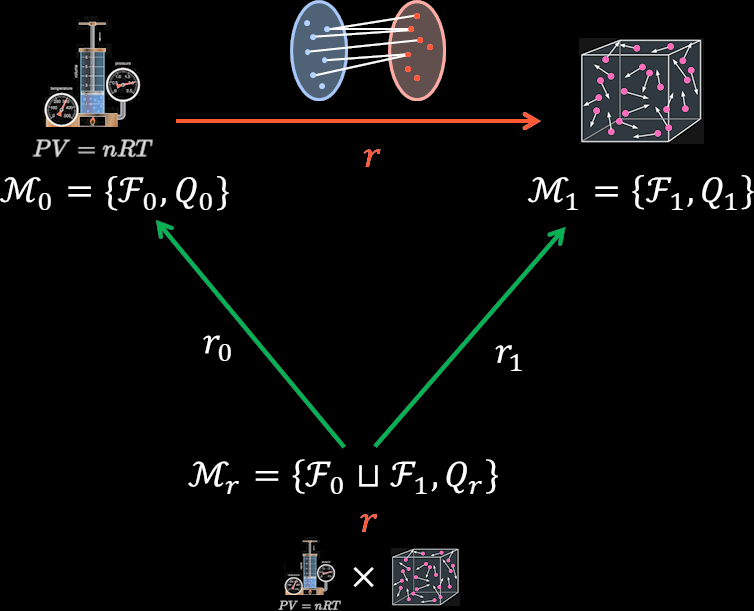# Underlying model of an imperfect morphism

We’ve already seen that if and are generalised models, with the relation a -preserving morphism between them, then there is an underlying model between them.

Since , is defined on ; indeed, it is non-zero on only. The underlying model has functions and to and , which push forward in a unique way—to and respectively. Essentially:

• There is an underlying reality of which and are different, consistent, facets.

Illustrated, for gas laws:## Underlying model of imperfect morphisms

But we’ve seen that relations need not be -preserving; there are weaker conditions that also form categories.

Indeed, even in the toy example above, the ideal gas laws and the “atoms bouncing around” model don’t have a -preserving morphism between them. The atoms bouncing model is more accurate, and the idea gas laws are just an approximation of these (for example, they ignore molar mass).

Let’s make the much weaker assumption that is -birelational—essentially that if any has non-zero -measure (i.e. ), then relates it to at least one other which also has non-zero -measure. Equivalently, if we ignore all elements with zero -measure, then and are surjective relations between what’s left. Then we have a more general underlying morphism result:

## Statement of the theorem

Let be a -birelational morphism between and , and pick any .

Then there exists a generalised model , with off of (this is not necessarily uniquely defined). This has natural functional morphisms and .

Those push forward to , such that for the distance metric defined on morphisms,

1. ,

2. .

By the definition of , this is the minimum we can get. The proof is in this footnote.

## Accuracy of models

If , we’re saying that is a correct model, and that is an approximation. Then the underlying model reflects this, with a true facet of the underlying model, and the closest-to-accurate facet that’s possible given the connection with . If , then it’s reversed: is an approximation, and a correct model. For between those two value, we see both and as approximations of the underlying reality .

## Measuring ontology change

This approach means that can be used to measure the extent of an ontology crisis.

Assume is a the initial ontology, and is the new ontology. Then might include entirely new situations, or at least unusual ones that were not normally thought about. The connects the old ontology with the new one: it details the crisis.

In an ontology crisis, there are several elements:

1. A completely different way of seeing the world.

2. The new and old ways result in similar predictions in standard situations.

3. The new way results in very different predictions in unusual situations.

4. The two ontologies give different probabilities to unusual situations.

The measure amalgamates points 2., 3., and 4. above, giving an idea of the severity of the ontology crisis in practice. A low might be because because the new and old ways have very similar predictions, or because the situations where they differ might be unlikely.

For point 1, the “completely different way of seeing the world”, this is about how features change and relate. The is indifferent to that, but we might measure this indirectly. We can already use a generalisation of mutual information to measure the relation between the distribution and the features . We could use that to measure the relation between , the features of , and , its probability distribution. Since is more strongly determined by , this could measure how hard it is to express in terms of .

1. Because is bi-relational, there is a such that is a -preserving morphism between and ; and furthermore . Let be an underlying model of this morphism.

Similarly, there is a such that is a -preserving morphism between and ; and furthermore . Let be an underlying model of this morphism. Note that and differ only in their and ; they have same feature sets and same worlds.

Then define as having . Then , so

Similarly, . ↩︎

2. This is a suggestion; there may be more direct ways of measuring this distance or complexity. ↩︎